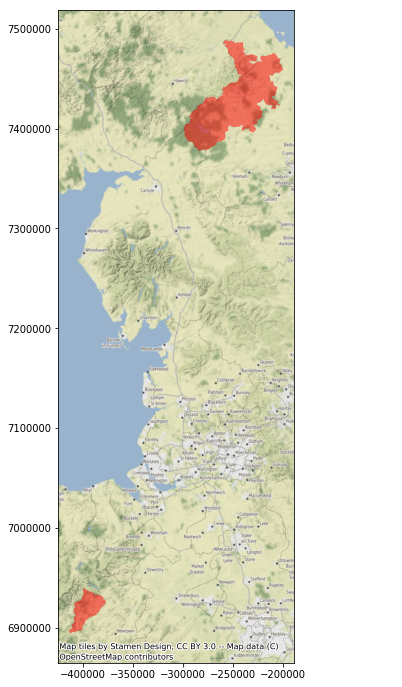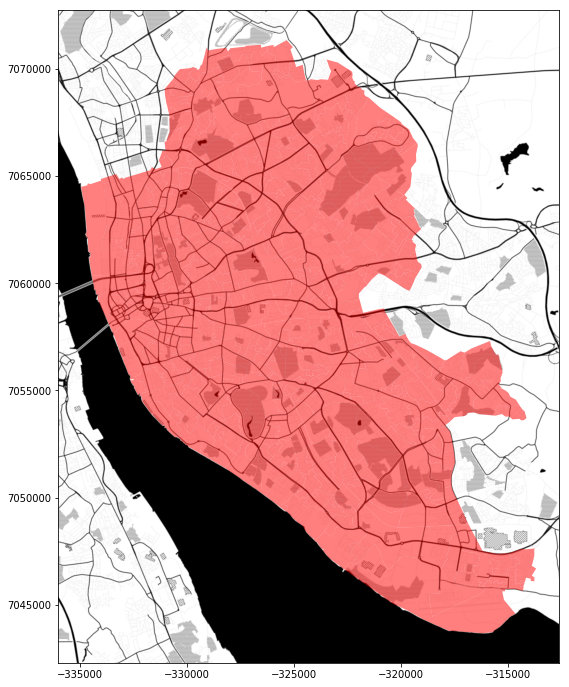# Reading LSOAs through the ONS API¶

In :
%matplotlib inline

import geopandas
import contextily
import requests
from io import BytesIO


In this short tutorial, we will explore how to pull down data from the ONS' Open Geography Portal. In particular, we will be working with 2011 LSOAs:

In :
from IPython.display import IFrame

site = ('http://geoportal.statistics.gov.uk/datasets/'\
'lower-layer-super-output-areas-december-2011-'\
'generalised-clipped-boundaries-in-england-and-wales/geoservice')
IFrame(site, 600, 400)

Out:

To read off the ONS API, we can use the base URL provided here:

In :
base_url = ("https://ons-inspire.esriuk.com/arcgis/rest/services/"\
"Census_Boundaries/"\
"Lower_Super_Output_Areas_December_2011_Boundaries/"\
"MapServer/2/query")


It is a standard REST API, which means we can pass the options as a set of parameters in a dictionary.

For example, let us ask for all the fields of the LSOAs with an area larger than 282,152,900 $m^2$, returned in LonLat (EPSG:4326) and in the GeoJSON format:

In :
params = {
'outFields': '*',
'where': 'st_area(shape) > 282152900',
'outSR': 4326,
'f': 'geojson'
}


NOTE: you can get all the details on which arguments are allowed here.

Now, we can pass the base URL with the query parameters to requests and let it do the hard work for us:

In :
r = requests.get(base_url, params=params)


At this point we have already downloaded the data (you can print it raw if you want calling r.content).

However, this is only half the job. We also need to turn that into a data structure that allows us to work with it in Python. Since the payload is returned as a GeoJSON, you can pass that to the file reader in geopandas tricking it to believe it's a file instead of a string with BytesIO:

In :
db = geopandas.read_file(BytesIO(r.content))


This operation builds a GeoDataFrame, with which we can work with in Python. for example, we can make a map that combines the polygons returned with a basemap pulled in from contextily:

In :
ax = db.to_crs(epsg=3857)\
.plot(alpha=0.5, color='red',
figsize=(12, 12))Now, just for fun, let us also demonstrate how you can make a slightly more sophisticated query. Exploring the table, we find that the lsoa11nm contains the name of the Local Authority District (LAD) each LSOA belongs to:

In :
db.head()

Out:
objectid lsoa11cd lsoa11nm lsoa11nmw st_area(shape) st_length(shape) geometry
0 26668 E01027373 Northumberland 007D Northumberland 007D 4.947698e+08 169371.260616 POLYGON ((-2.146180530748926 55.46365862427746...
1 26670 E01027375 Northumberland 003B Northumberland 003B 4.127454e+08 153629.193118 POLYGON ((-2.289024616370855 55.64350755966522...
2 26794 E01027503 Northumberland 019C Northumberland 019C 6.837843e+08 135783.112983 POLYGON ((-2.329234461936109 55.36792102662705...
3 33283 W01000452 Powys 004B Powys 004B 2.827696e+08 101339.899414 POLYGON ((-3.584752415641088 52.76577014395649...

We can use this then to query polygons from a single LAD, such as Liverpool (note this uses standard SQL):

In :
params = {
'where': "lsoa11nm LIKE '%Liverpool%'",
'outSR': 4326,
'f': 'geojson'
}


And we can now pull the data and turn it into a GeoDataFrame in the same way as above:

In :
r = requests.get(base_url, params=params)



Let's check the results:

In :
ax = db.to_crs(epsg=3857)\
.plot(alpha=0.5, color='red',
figsize=(12, 12))
url=contextily.sources.ST_TONER_BACKGROUND);🎉

## Connecting ONS geometries with IMD¶

In :
import pandas

imd_url = ('https://assets.publishing.service.gov.uk'
'/file/467764/File_1_ID_2015_Index_of_Multiple_Deprivation.xlsx')

Out:
LSOA code (2011) LSOA name (2011) Local Authority District code (2013) Local Authority District name (2013) Index of Multiple Deprivation (IMD) Rank (where 1 is most deprived) Index of Multiple Deprivation (IMD) Decile (where 1 is most deprived 10% of LSOAs)
In :
db_imd = db.join(imd.set_index('LSOA code (2011)')\
.iloc[:, -1:]\
.rename(columns={('Index of Multiple Deprivation (IMD) Decile '\
'(where 1 is most deprived 10% of LSOAs)'): 'score'}),
on='lsoa11cd')
db_imd.info()

<class 'geopandas.geodataframe.GeoDataFrame'>
RangeIndex: 298 entries, 0 to 297
Data columns (total 3 columns):
lsoa11cd    298 non-null object
geometry    298 non-null object
score       298 non-null int64
dtypes: int64(1), object(2)
memory usage: 7.1+ KB

In :
ax = db_imd.to_crs(epsg=3857)\
.plot(column='score', scheme='quantiles',
alpha=0.75, figsize=(12, 12))

/opt/conda/lib/python3.7/site-packages/mapclassify/classifiers.py:95: UserWarning: Warning: Not enough unique values in array to form k classes